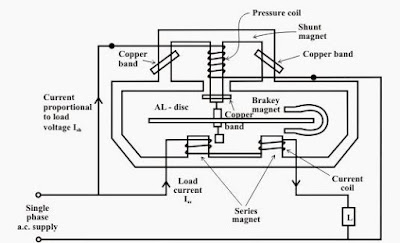# Three Phase Energy Meter – Construction & Working

0
364

## Counting system:

#### These can be seen in the diagram given below:#### A 3-phase induction motor has same four systems but they are arranged in a different way as shown in the figure given below.### Working on three-phase energy meter:

#### where

Pi = Real power being used at that point in time in kW
T = Time (in seconds) for the disc to rotate through the N rotations or part of a rotation
N = The Number of full rotations counted.
R = The number of revolutions per Kilowatt hour (rev/kWh) of the meter being used.

#### In this way, we use a three-phase energy meter to calculate the energy consumed by the load.

READ HERE  Capacitor Types: Symbols, Functions, Applications, and FAQs